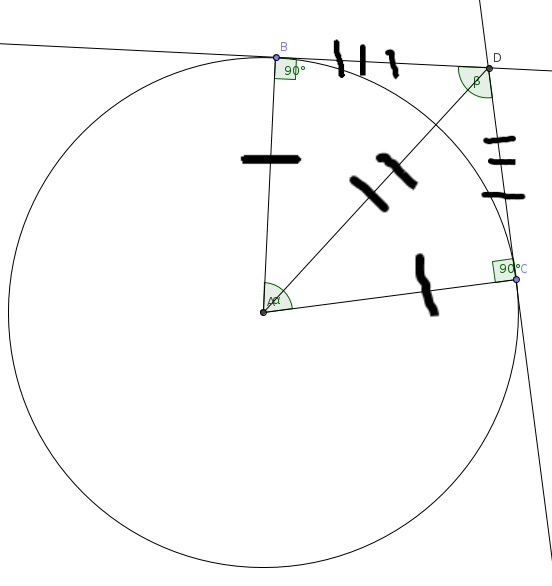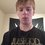# Area Between Two Tangents on a Circle Equation

$a_{t}=\dfrac{r^{2}}{\tan\left ( \dfrac{\beta}{2} \right )}- \dfrac{\pi \cdot r^{2}\alpha}{360}$

Here is an equation I derived in a simple manner for finding the area between two tangents on a circle in terms of the radius if given their angle of intersection, as shown above.

In accordance with this diagram:We can prove with SSS or SAS that the two triangles are congruent. Here, beta is the angle of intersection and beta is the supplement of alpha. All I am doing is finding the areas of both triangles and subtracting from it the area section of our circle, whatever remains is the area between the curves. Nothing fancy, but it is nice to generalize something, and it might come in handy for someone somewhere.Note by Drex Beckman
5 years, 5 months ago

This discussion board is a place to discuss our Daily Challenges and the math and science related to those challenges. Explanations are more than just a solution — they should explain the steps and thinking strategies that you used to obtain the solution. Comments should further the discussion of math and science.

When posting on Brilliant:

• Use the emojis to react to an explanation, whether you're congratulating a job well done , or just really confused .
• Ask specific questions about the challenge or the steps in somebody's explanation. Well-posed questions can add a lot to the discussion, but posting "I don't understand!" doesn't help anyone.
• Try to contribute something new to the discussion, whether it is an extension, generalization or other idea related to the challenge.

MarkdownAppears as
*italics* or _italics_ italics
**bold** or __bold__ bold
- bulleted- list
• bulleted
• list
1. numbered2. list
1. numbered
2. list
Note: you must add a full line of space before and after lists for them to show up correctly
paragraph 1paragraph 2

paragraph 1

paragraph 2

[example link](https://brilliant.org)example link
> This is a quote
This is a quote
    # I indented these lines
# 4 spaces, and now they show
# up as a code block.

print "hello world"
# I indented these lines
# 4 spaces, and now they show
# up as a code block.

print "hello world"
MathAppears as
Remember to wrap math in $$ ... $$ or $ ... $ to ensure proper formatting.
2 \times 3 $2 \times 3$
2^{34} $2^{34}$
a_{i-1} $a_{i-1}$
\frac{2}{3} $\frac{2}{3}$
\sqrt{2} $\sqrt{2}$
\sum_{i=1}^3 $\sum_{i=1}^3$
\sin \theta $\sin \theta$
\boxed{123} $\boxed{123}$

Sort by:

This formula would look a little neater if you'd have expressed all angles in radians.

- 5 years, 5 months ago

@Deeparaj Bhat Right. So I guess: $\frac {r^{2}}{tan (\frac{\beta}{2})}- \frac{r^{2}\alpha} {2}$. You are right, it looks a lot nicer. I tried also getting a common denominator, but that looks even worse than using degrees. Thank you, sir.

- 5 years, 5 months ago

@Drex Beckman On plugging in $\beta$, the formula becomes,

$\frac{r^2}{2}\cdot(\tan{\frac{\alpha}{2}}-\frac{\alpha}{2})$

- 5 years, 4 months ago

@Deeparaj Bhat Can you please tell me how you derived that? Sorry, I'm lacking in maths. Is there an identity you used? Also, am I doing something wrong? I tried this with my question: $\frac{r^{2}}{2}(tan (\frac {\alpha}{2})-\frac{\alpha}{2})$ where $\alpha = 0.479965 radians$, and I got an answer of $\approx 0.00236$, which is exactly half the answer. I think it should be r squared itself, not divided by two, right? I tried this with different r, too. Thanks for your help, it is really appreciated!

- 5 years, 4 months ago

Sorry, my bad. It was supposed to be $r^2(\tan{(\frac{\alpha}{2})}-\frac{\alpha}{2})$, like you said.

I used the following two facts for deriving it:

$\alpha+\beta=\pi$ $\tan{(\frac{\pi}{2}-x)}=\cot{x}$

The first follows by the fact that sum of angles in a quadrilateral ( here ABCD) is $2\pi$ and since $\angle ACD=\angle ABD=\frac{\pi}{2}$

The second is a well known identity which you can find in one of wikis on Brilliant.

- 5 years, 4 months ago

Okay, I get it now. I don't really remember lots of trig identities, so I should obviously review them. :P But you did a great job simplifying it. It really does look a lot more beautiful that way. Thanks! :D

- 5 years, 4 months ago

In fact, most of the level 5 geometry problems can be solved (almost) exclusively by using trig identities!

- 5 years, 4 months ago

Seriously?! Good to know, man. I learned them at school a few years ago, and never used them again. But that was before I thought mathematics was fun. Too bad I didn't get interested in it earlier, because I am way far behind people like you, also I think most of the people on here are smarter than me. xD

- 5 years, 4 months ago